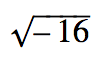Real Numbers

My Definition

Key Characteristics

The following are true of real numbers:

• Real numbers include both rational numbers and irrational numbers.

• All real numbers can be plotted on a number line.

Example

Use the link below to further study examples of real numbers.

Real Numbers Activity 1
Real Numbers Activity 2

Non-exampleThis is not a real number. It is an imaginary number.

The value cannot be plotted on a number line.

TEKS: 6(2)(A), 8(2)(A)